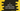# C program to add two numbers using their addresses### C program to add two numbers using their addresses :

In this tutorial, we will learn how to add two numbers using addresses in C programming language. Mainly we will learn how to access the values of one address in C. Let’s take a look into the algorithm first :

### Algorithm :

1. First ask the user to input the numbers and store them in two integer variables.
2. Get the address of both numbers and store them in two different variables.
3. Read the numbers using the address .
4. Add both of them and store it in a different variable.
5. Print out the result.

### C program :

``````#include <stdio.h>

int main()
{
//1
int first, second;

//2
int *firstAddress, *secondAddress;

//3
int sum;

//4
printf("Enter the first and second number : \n");

//5
scanf("%d %d", &first, &second);

//6
firstAddress = &first;
secondAddress = &second;

//7
sum = *firstAddress + *secondAddress;

//8
printf("The sum of %d and %d is %d \n", first, second, sum);
return 0;
}``````

### Explanation :

_The commented numbers in the above program denotes the step number below : _

1. Create two variables to store the first and second number : first and second.
2. Create two pointer variables to store the address of the numbers : firstAddress and secondAddress.
3. Create one variable to save the sum of these numbers : sum.
4. Ask the user to enter the first and the second number.
5. Using scanf store the user input values in first and second variables.
6. Store the address of variable first and second in firstAddress and secondAddress.
7. Get the values containing inside these addresses and add them. Use *addressVariable to get the content of the address addressVariable. Store the sum in sum variable.
8. Print the first number, second number and sum of both numbers to the user.

### Sample output :

``````Enter the first and second number :
12 45
The sum of 12 and 45 is 57``````